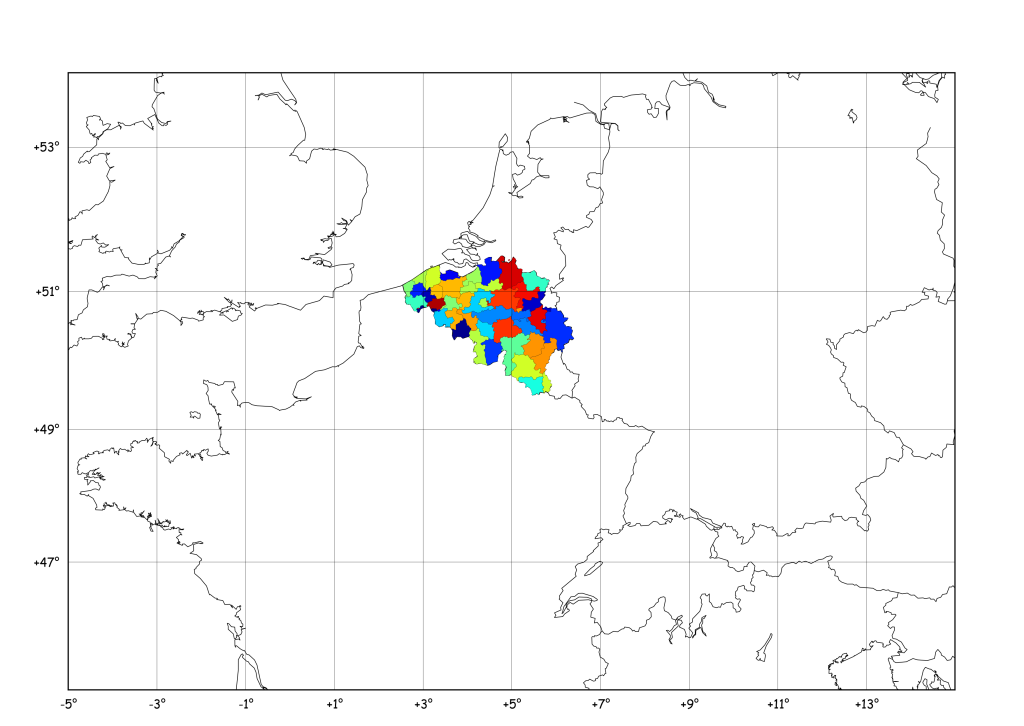## Matplotlib Basemap tutorial 10: Shapefiles Unleached, continued

Following the comments on this year-old post I’ve had a look at pyshp which seems a little more maintained (the last line in the changelog is 1 year old…), and it is indeed a quite nice piece of code. I…

## New on Géophysique.be: Gallery

Everyone used to search for code snippets on the web know the importance of a “Gallery” linking to all examples and tutorials… So, here is my really ugly Gallery :

## Matplotlib Basemap tutorial 09: Drawing circles

In the previous tutorial, I defined a “shoot” method to compute the landing point of a shoot from one point, to a given azimuth and distance. Using this logic, it’s possible to find the points situated at a given distance…

## Matplotlib Basemap tutorial 08: Shooting Great Circles

Following a question from Ricardo Gama (see his comment), I’ve prepared this new tutorial. He wondered if Basemap has a function similar to the track1 function in matlab (you know, that crappy costly thing…)… Here is what I obtained :…

## Matplotlib Basemap: Tell me what you need !

Dear visitors, I’m always searching new ideas of preparing new tutorials for things doable with Basemap, but I’d like these examples to be as useful as possible, which means : If you have something you would like to appear here,…

## Matplotlib Basemap tutorial 07: Shapefiles unleached

New version here Following a question in the matplotlib mailing list, I dug inside the code of readshapefile, in order to gain power : The goal: The data: http://www.gadm.org/ saved inside a new “borders/” folder ! The idea: Opening a…

## Matplotlib Basemap tutorial 06: Real Case pie charts

Here is a new tutorial that will include “a bit of all” tutorials previously published on this blog and some new cool stuff to play with ! Idea: Find some resources on the Internet and plot them on the map…

## Matplotlib Basemap tutorial 05 : Adding some pie charts !

Hi folkes, Following a question by Gui on this website, I checked the “how to” draw pie charts on a basemap plot ! Here below I generalise the example given by Manuel Metz on the matplotlib website. The idea is…

## Matplotlib Basemap tutorial 04 : using inside_poly() to select data !

# remember to define the ax instance : ax = plt.subplot(111) zone = “Roetgen – Monschau” x,y = m(6.15,50.41) x2,y2 = m(6.59,50.67) x3,y3 = m(6.34,50.855) x4,y4 = m(5.83,50.65) data = np.array(([x,x2,x3,x4], [y,y2,y3,y4])) p = Polygon(data.T,edgecolor=’red’,linewidth=1.5,facecolor=’none’) ax.add_artist(p) Note that we have…

## Matplotlib Basemap tutorial 03 : Masked arrays & Zoom

Here, we will focus on adding a “zoom box” on the top left corner of the plot. But before that, we will mask a part of the earthquakes, in order to have a “cleaner” map ! This is achieved by…

## Matplotlib Basemap tutorial 02 : Let’s add some earthquakes !

Now, let’s imagine we have a dataset containing latitude/longitudes of earthquakes, plus their depth and magnitude. Of course, you don’t always have this dataset available, so let’s build a fake one : import numpy as np lon = np.random.random_integers(11,79,1000)/10. lat…

## Matplotlib Basemap tutorial 01 : Your first map

# # BaseMap example by geophysique.be # tutorial 01 from mpl_toolkits.basemap import Basemap import matplotlib.pyplot as plt import numpy as np fig = plt.figure(figsize=(11.7,8.3)) #Custom adjust of the subplots plt.subplots_adjust(left=0.05,right=0.95,top=0.90,bottom=0.05,wspace=0.15,hspace=0.05) ax = plt.subplot(111) #Let’s create a basemap around Belgium m…